﻿ C Program: Search an element in a circular linked list - w3resource

# C Exercises: Search an element in a circular linked list

## C Doubly Linked List : Exercise-19 with Solution

Write a program in C to search an element in a circular linked list.

Pictorial Presentation: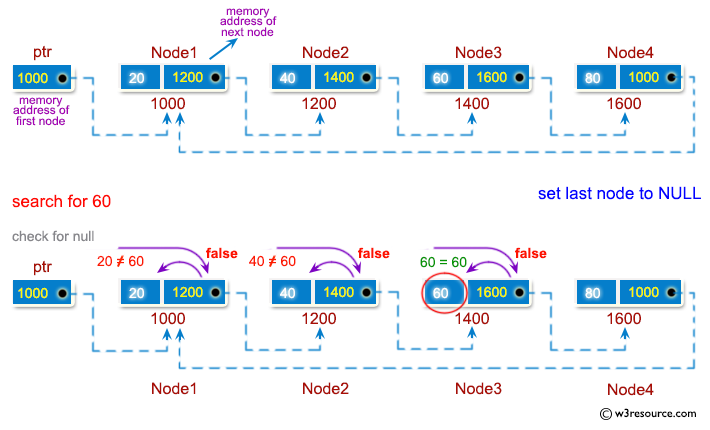Sample Solution:

C Code:

``````#include <stdio.h>
#include <stdlib.h>

struct node {
int num;
struct node * nextptr;
}*stnode,*ennode;

void ClListcreation(int n);
int FindElement(int FindElem, int n);
void displayClList();

int main()
{
int n,m;
int i,FindElem,FindPlc;
stnode = NULL;
ennode = NULL;
printf("\n\n Circular Linked List : Search an element in a circular linked list :\n");
printf("-------------------------------------------------------------------------\n");

printf(" Input the number of nodes : ");
scanf("%d", &n);
m=n;

ClListcreation(n);
displayClList();
printf(" Input the element you want to find : ");
scanf("%d", &FindElem);

FindPlc=FindElement(FindElem,m);
if(FindPlc<n)
printf(" Element found at node %d \n\n",FindPlc);
else
printf(" This element does not exists in linked list.\n\n");

return 0;
}

void ClListcreation(int n)
{
int i, num;
struct node *preptr, *newnode;

if(n >= 1)
{
stnode = (struct node *)malloc(sizeof(struct node));

printf(" Input data for node 1 : ");
scanf("%d", &num);
stnode->num = num;
stnode->nextptr = NULL;
preptr = stnode;
for(i=2; i<=n; i++)
{
newnode = (struct node *)malloc(sizeof(struct node));
printf(" Input data for node %d : ", i);
scanf("%d", &num);
newnode->num = num;
newnode->nextptr = NULL;	// next address of new node set as NULL
preptr->nextptr = newnode;	// previous node is linking with new node
preptr = newnode;   		// previous node is advanced
}
preptr->nextptr = stnode; 		//last node is linking with first node
}
}

int FindElement(int FindElem, int a)
{
int ctr=1;
ennode=stnode;
while(ennode->nextptr!=NULL)
{
if(ennode->num==FindElem)
break;
else
ctr++;
ennode=ennode->nextptr;
if (ctr==a+1)
break;
}
return ctr;
}

void displayClList()
{
struct node *tmp;
int n = 1;

if(stnode == NULL)
{
printf(" No data found in the List yet.");
}
else
{
tmp = stnode;
printf("\n\n Data entered in the list are :\n");

do {
printf(" Data %d = %d\n", n, tmp->num);

tmp = tmp->nextptr;
n++;
}while(tmp != stnode);
}
}
```
```

Sample Output:

``` Circular Linked List : Search an element in a circular linked list :
-------------------------------------------------------------------------
Input the number of nodes : 3
Input data for node 1 : 2
Input data for node 2 : 5
Input data for node 3 : 9

Data entered in the list are :
Data 1 = 2
Data 2 = 5
Data 3 = 9
Input the element you want to find : 5
Element found at node 2
```

Flowchart :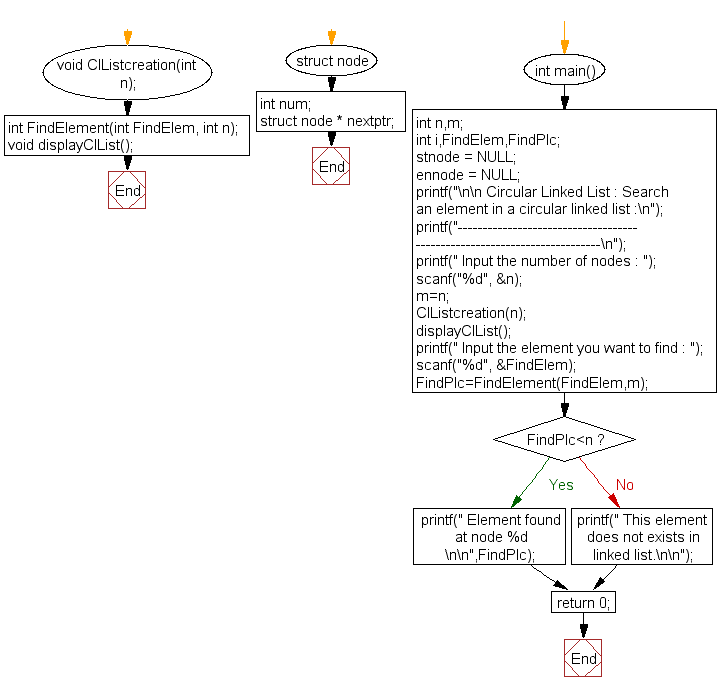ClListcreation() :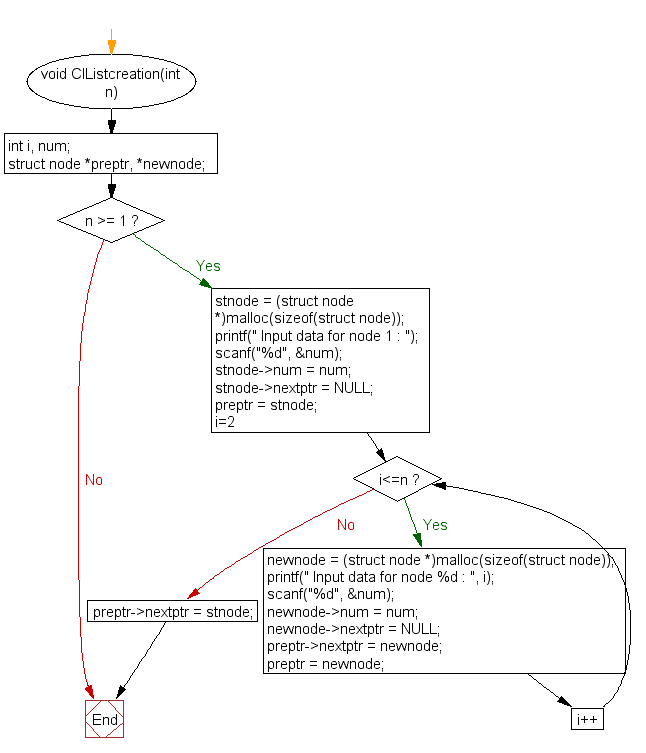FindElement() :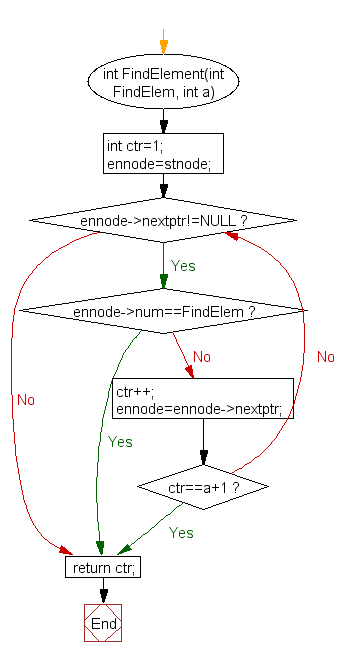displayClList() :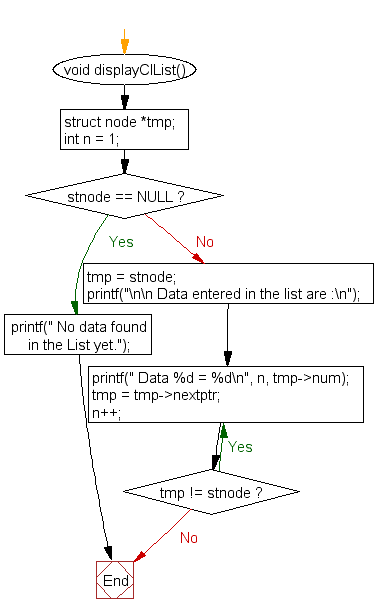C Programming Code Editor:

What is the difficulty level of this exercise?

Test your Programming skills with w3resource's quiz.

﻿